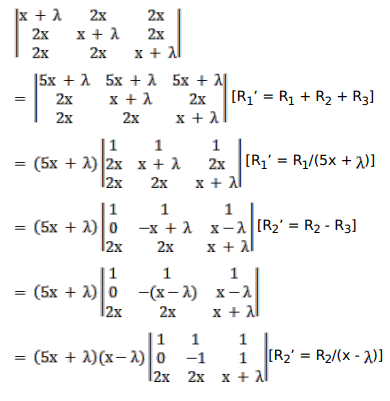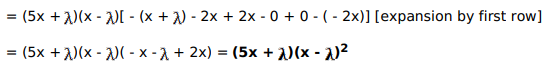Deepak Scored 45->99%ile with Bounce Back Crack Course. You can do it too!

# Using properties of determinants prove that:

Question:

Using properties of determinants prove that:

$\left|\begin{array}{ccc}x+\lambda & 2 x & 2 x \\ 2 x & x+\lambda & 2 x \\ 2 x & 2 x & x+\lambda\end{array}\right|=(5 x+\lambda)(\lambda-x)^{2}$

Solution: